﻿ 一类快慢耦合Duffing-van der Pol系统的平衡点分析

一类快慢耦合Duffing-van der Pol系统的平衡点分析Equilibrium Analysis of a Class of Fast-Slow Coupled Duffing-van der Pol System

Abstract: This paper studies the equilibrium point problem of a class of fast-slow coupled Duffing-van der Pol system with the coexistence of quintic term and cubic term. Quintic equation of one variable is obtained by solving the equilibrium points of the system. By using the method of reducing the order of n degree equation of one variable, the order of the equilibrium equation is reduced from five to four. When the equilibrium equation is reduced to the quartic, the coefficient of the constant term is calculated to be zero, so it can be directly reduced to the cubic equation. Morigane formula is used to solve the cubic equation. The distribution of the solution of the equilibrium equation on the F-u1 plane is obtained by synthesizing these four cases.

1. 引言

Duffing方程和van der Pol方程都是典型的非线性振动系统，已有不少学者对这两类方程的动力学行为进行研究  - 。本文主要研究了一类5次项和3次项共存的快慢耦合Duffing-van der Pol系统的平衡点   问题。我们在分析平衡点时，会遇到一元n次方程。

2. 数学模型与平衡点方程

$\left\{\begin{array}{l}\stackrel{˙}{x}=y,\\ \stackrel{˙}{y}={u}_{1}x+{u}_{2}y+{x}^{3}+{x}^{5}+a{x}^{2}y+b{x}^{4}y.\end{array}$ (1)

$\left\{\begin{array}{l}\stackrel{˙}{x}=y,\\ \stackrel{˙}{y}={u}_{1}x+{u}_{2}y+{x}^{3}+{x}^{5}+a{x}^{2}y+b{x}^{4}y+f\mathrm{cos}\omega t,\end{array}$ (2)

$\left\{\begin{array}{l}\stackrel{˙}{x}=y,\\ \stackrel{˙}{y}={u}_{1}x+{u}_{2}y+{x}^{3}+{x}^{5}+a{x}^{2}y+b{x}^{4}y+F.\end{array}$ (3)

$\left\{\begin{array}{l}y=0,\\ {u}_{1}x+{x}^{3}+{x}^{5}+F=0.\end{array}$ (4)

${x}^{5}=-{x}^{3}-{u}_{1}x-F.$ (5)

3. 一元 次方程的降次解法分析

${y}^{\left(5\right)}=-{y}^{\left(3\right)}-{u}_{1}{y}^{\left(1\right)}-Fy.$ (6)

$y\left(t\right)={\sum }_{j=1}^{5}{C}_{j}{Y}_{j}\left(t\right),$ (7)

${Y}_{j}\left(t\right)={t}^{j-1}+{\sum }_{m=1}^{\infty }{}^{5}{\int }_{0}^{t}\left(-{y}_{j\left(m-1\right)}^{\left(3\right)}-{u}_{1}{y}_{j\left(m-1\right)}^{\left(1\right)}-F{y}_{j\left(m-1\right)}\right){\left(\text{d}t\right)}^{5}\left(j=1,2,\cdots ,n\right).$ (8)

${\text{e}}^{\xi t}={\sum }_{j=1}^{5}{C}_{j}{Y}_{j}\left(t\right).$ (9)

$t=0$$t=1$$t=2$$t=3$$t=4$ 代入方程9，得到

${C}_{1}=1,{\text{e}}^{\xi }={\sum }_{j=1}^{5}{C}_{j}{Y}_{j}\left(1\right),\cdots ,{\text{e}}^{4\xi }={\sum }_{j=1}^{5}{C}_{j}{Y}_{j}\left(4\right).$ (10)

${Y}_{1}\left(1\right)=1+\left(-F\right)+{\left(-F\right)}^{2}+\cdots +{\left(-F\right)}^{m}+\cdots =1-\frac{F{\mathrm{lim}}_{m\to \infty }\left[1-{\left(-F\right)}^{m}\right]}{1+F}$ (11)

$\begin{array}{c}{Y}_{2}\left(1\right)=1+\left(-{u}_{1}-\frac{1}{2}F\right)+\left(-{u}_{1}-\frac{1}{2}F\right)\left(-F\right)+\cdots +\left(-{u}_{1}-\frac{1}{2}F\right){\left(-F\right)}^{m-1}+\cdots \\ =1-\left(-{u}_{1}-\frac{1}{2}F\right){Y}_{1}\left( 1 \right)\end{array}$

$⋮$

$\begin{array}{c}{b}_{0}=2\left[{Y}_{2}\left(1\right){Y}_{3}\left(1\right){Y}_{4}\left(2\right){Y}_{5}\left(3\right)-{Y}_{2}\left(1\right){Y}_{3}\left(1\right){Y}_{5}\left(2\right){Y}_{4}\left(3\right)+{Y}_{2}\left(1\right){Y}_{5}\left(1\right){Y}_{3}\left(2\right){Y}_{4}\left(3\right)\\ \text{\hspace{0.17em}}\text{\hspace{0.17em}}-{Y}_{2}\left(1\right){Y}_{5}\left(1\right){Y}_{4}\left(2\right){Y}_{3}\left(3\right)-{Y}_{3}\left(1\right){Y}_{4}\left(1\right){Y}_{2}\left(2\right){Y}_{5}\left(3\right)+{Y}_{3}\left(1\right){Y}_{4}\left(1\right){Y}_{5}\left(2\right){Y}_{2}\left(3\right)\\ \text{\hspace{0.17em}}\text{\hspace{0.17em}}+{Y}_{4}\left(1\right){Y}_{5}\left(1\right){Y}_{2}\left(2\right){Y}_{3}\left(3\right)-{Y}_{4}\left(1\right){Y}_{5}\left(1\right){Y}_{3}\left(2\right){Y}_{2}\left(3\right)\right],\end{array}$

$\begin{array}{c}{b}_{1}=\frac{2}{{b}_{0}}\left[{Y}_{3}\left(1\right){Y}_{2}\left(2\right){Y}_{4}\left(3\right){Y}_{5}\left(4\right)-{Y}_{3}\left(1\right){Y}_{2}\left(2\right){Y}_{5}\left(3\right){Y}_{4}\left(4\right)-{Y}_{3}\left(1\right){Y}_{4}\left(2\right){Y}_{2}\left(3\right){Y}_{5}\left(4\right)\\ \text{\hspace{0.17em}}\text{\hspace{0.17em}}+{Y}_{3}\left(1\right){Y}_{4}\left(2\right){Y}_{5}\left(3\right){Y}_{2}\left(4\right)+{Y}_{3}\left(1\right){Y}_{5}\left(2\right){Y}_{2}\left(3\right){Y}_{4}\left(4\right)-{Y}_{3}\left(1\right){Y}_{5}\left(2\right){Y}_{4}\left(3\right){Y}_{2}\left(4\right)\\ \text{\hspace{0.17em}}\text{\hspace{0.17em}}+{Y}_{5}\left(1\right){Y}_{2}\left(2\right){Y}_{3}\left(3\right){Y}_{4}\left(4\right)-{Y}_{5}\left(1\right){Y}_{2}\left(2\right){Y}_{4}\left(3\right){Y}_{3}\left(4\right)-{Y}_{5}\left(1\right){Y}_{3}\left(2\right){Y}_{2}\left(3\right){Y}_{4}\left(4\right)\\ \text{\hspace{0.17em}}\text{\hspace{0.17em}}+{Y}_{5}\left(1\right){Y}_{3}\left(2\right){Y}_{4}\left(3\right){Y}_{2}\left(4\right)+{Y}_{5}\left(1\right){Y}_{4}\left(2\right){Y}_{2}\left(3\right){Y}_{3}\left(4\right)-{Y}_{5}\left(1\right){Y}_{4}\left(2\right){Y}_{3}\left(3\right){Y}_{2}\left(4\right)\right],\end{array}$

$\begin{array}{c}{b}_{2}=\frac{2}{{b}_{0}}\left[{Y}_{2}\left(1\right){Y}_{3}\left(1\right){Y}_{4}\left(3\right){Y}_{5}\left(4\right)-{Y}_{2}\left(1\right){Y}_{3}\left(1\right){Y}_{5}\left(3\right){Y}_{4}\left(4\right)+{Y}_{2}\left(1\right){Y}_{5}\left(1\right){Y}_{3}\left(3\right){Y}_{4}\left(4\right)\\ \text{\hspace{0.17em}}\text{\hspace{0.17em}}-{Y}_{2}\left(1\right){Y}_{5}\left(1\right){Y}_{4}\left(3\right){Y}_{3}\left(4\right)-{Y}_{3}\left(1\right){Y}_{4}\left(1\right){Y}_{2}\left(3\right){Y}_{5}\left(4\right)+{Y}_{3}\left(1\right){Y}_{4}\left(1\right){Y}_{5}\left(3\right){Y}_{2}\left(4\right)\\ \text{\hspace{0.17em}}\text{\hspace{0.17em}}+{Y}_{4}\left(1\right){Y}_{5}\left(1\right){Y}_{2}\left(3\right){Y}_{3}\left(4\right)-{Y}_{4}\left(1\right){Y}_{5}\left(1\right){Y}_{3}\left(3\right){Y}_{2}\left(4\right)\right],\end{array}$

$\begin{array}{c}{b}_{3}=\frac{2}{{b}_{0}}\left[{Y}_{2}\left(1\right){Y}_{3}\left(1\right){Y}_{4}\left(2\right){Y}_{5}\left(4\right)-{Y}_{2}\left(1\right){Y}_{3}\left(1\right){Y}_{5}\left(2\right){Y}_{4}\left(4\right)+{Y}_{2}\left(1\right){Y}_{5}\left(1\right){Y}_{3}\left(2\right){Y}_{4}\left(4\right)\\ \text{\hspace{0.17em}}\text{\hspace{0.17em}}-{Y}_{2}\left(1\right){Y}_{5}\left(1\right){Y}_{4}\left(2\right){Y}_{3}\left(4\right)-{Y}_{3}\left(1\right){Y}_{4}\left(1\right){Y}_{2}\left(2\right){Y}_{5}\left(4\right)+{Y}_{3}\left(1\right){Y}_{4}\left(1\right){Y}_{5}\left(2\right){Y}_{2}\left(4\right)\\ \text{\hspace{0.17em}}\text{\hspace{0.17em}}+{Y}_{4}\left(1\right){Y}_{5}\left(1\right){Y}_{2}\left(2\right){Y}_{3}\left(4\right)-{Y}_{4}\left(1\right){Y}_{5}\left(1\right){Y}_{3}\left(2\right){Y}_{2}\left(4\right)\right],\end{array}$

$c=0.$

${z}^{4}+{b}_{3}{z}^{3}+{b}_{2}{z}^{2}+{b}_{1}z+c=0.$ (12)

${z}^{3}+{b}_{3}{z}^{2}+{b}_{2}z+{b}_{1}=0.$ (13)

${z}_{1}$${z}_{2}$${z}_{3}$${z}_{4}$ 是方程(12)的4个根，那么 $\mathrm{ln}{z}_{1}$$\mathrm{ln}{z}_{2}$$\mathrm{ln}{z}_{3}$$\mathrm{ln}{z}_{4}$ 就是方程(5)的4个根， $-\left(\mathrm{ln}{z}_{1}+\mathrm{ln}{z}_{2}+\mathrm{ln}{z}_{3}+\mathrm{ln}{z}_{4}\right)$ 是第5个根。

4. 平衡点分析

$\begin{array}{l}A={b}_{3}^{2}-3{b}_{2},\\ B={b}_{2}{b}_{3}-9{b}_{1},\\ C={b}_{2}^{2}-3{b}_{1}{b}_{3},\\ \text{Δ}={B}^{2}-4AC.\end{array}$ (14)

4.1. 情形①： $A=B=0$

$A=B=0$，即

${b}_{3}^{2}-3{b}_{2}=0.$ (15)

${b}_{2}{b}_{3}-9{b}_{1}=0.$ (16)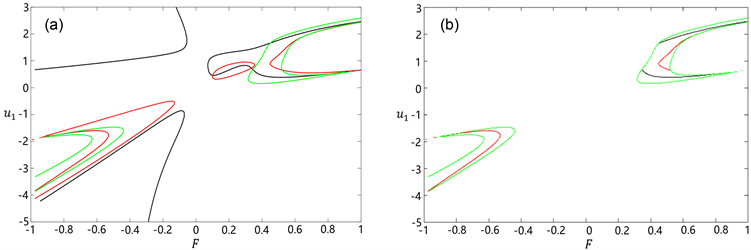Figure 1. The range of z on the F-u1 plane under case ①: (a) No condition z > 0; (b) Have condition z > 0

4.2. 情形②： $\text{Δ}>0$

$\text{Δ}>0$，即

${\left({b}_{2}{b}_{3}-9{b}_{1}\right)}^{2}-4\left({b}_{3}^{2}-3{b}_{2}\right)\left({b}_{2}^{2}-3{b}_{1}{b}_{3}\right)>0$ (17)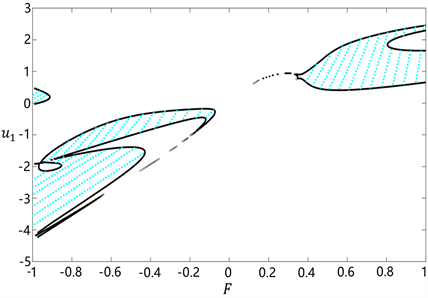Figure 2. Δ = 0 on the F-u1 plane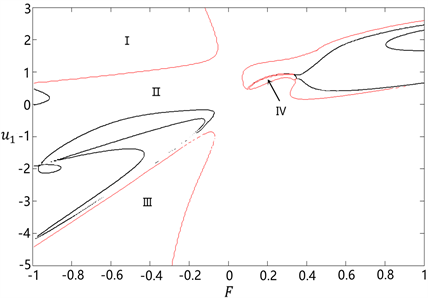Figure 3. The range of z on the F-u1 plane under case ②

4.3. 情形③： $\text{Δ}=0$

$\text{Δ}=0$，即

${\left({b}_{2}{b}_{3}-9{b}_{1}\right)}^{2}-4\left({b}_{3}^{2}-3{b}_{2}\right)\left({b}_{2}^{2}-3{b}_{1}{b}_{3}\right)=0$ (18)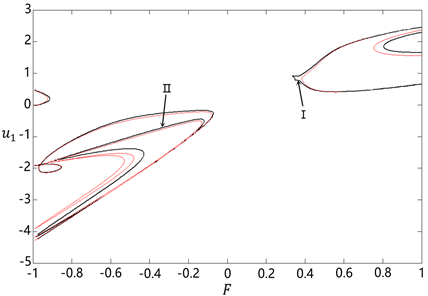Figure 4. The range of z on the F-u1 plane under case ③

4.4. 情形④： $\text{Δ}<0$

$\text{Δ}<0$，即

${\left({b}_{2}{b}_{3}-9{b}_{1}\right)}^{2}-4\left({b}_{3}^{2}-3{b}_{2}\right)\left({b}_{2}^{2}-3{b}_{1}{b}_{3}\right)<0$ (19)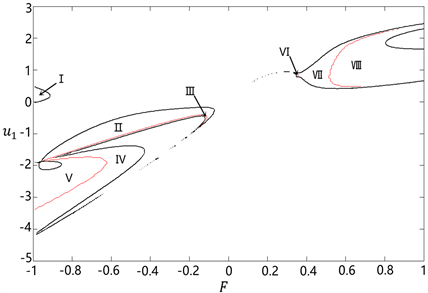Figure 5. The range of z on the F-u1 plane under case ④

4.5. 解个数分析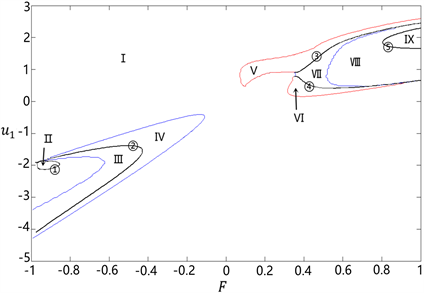Figure 6. Number distribution of x

5. 结论

NOTES

*通讯作者。

 Xu, Y., et al. (2011) Stochastic Bifurcations in a Bistable Duffing-Van der Pol Oscillator with Colored Noise. Physical Review E, 83, Article ID: 056215.
https://doi.org/10.1103/PhysRevE.83.056215

 Li, C., et al. (2013) Response Probability Density Functions of Duffing-Van der Pol Vibro-Impact System under Correlated Gaussian White Noise Excitations. Physics A, 392, 1269-1279.
https://doi.org/10.1016/j.physa.2012.11.053

 Zhang, C., et al. (2014) On Two-Parameter Bifurcation Analysis of Switched System Composed of Duffing and van der Pol Oscillators. Communications in Nonlinear Science and Numerical Simulation, 19, 750-757.
https://doi.org/10.1016/j.cnsns.2013.06.028

 Zhou, L. and Chen, F. (2014) Chaotic Motions of the Duffing-Van der Pol Oscillator with External and Parametric Excitations. Shock and Vibration, 2014, Article ID: 131637.
https://doi.org/10.1155/2014/131637

 Kumar, P., Narayanan, S. and Gupta, S. (2016) Stochastic Bifurcations in a Vibro-Impact Duffing-Van der Pol Oscillator. Nonlinear Dynamics, 85, 439-452.
https://doi.org/10.1007/s11071-016-2697-1

 Li, S., Niu, J. and Li, X. (2018) Primary Resonance of Fractional-Order Duffing-van der Pol Oscillator by Harmonic Balance Method. Chinese Physics B, 27, 211-216.
https://doi.org/10.1088/1674-1056/27/12/120502

 高发宝, 王永青. 一类五次非线性系统的全局动力学[J]. 动力系统与控制, 2020, 9(4): 207-213.

 陈乐颀. 一类带有超越功能反应的捕食者-食饵系统的平衡点分析[J]. 内江师范学院学报, 2020, 35(4): 34-37.

 张远达. 浅谈高次方程[M]. 武汉: 湖北教育出版社, 1983.

 林文业. 现代数学与量子力学[M]. 郑州: 郑州大学出版社, 2010.

 秦小兵. 关于高次方程根的研究[J]. 基础教育课程, 2017(10): 65-67.

 姜海馨, 姜玉秋. 论一元高次方程的近似求解[J]. 文存阅刊, 2017(1): 37, 39.

 范盛金. 一元三次方程的新求根公式与新判别法[J]. 海南师范学院学报, 1989(2): 91-98.

 刘延彬, 陈予恕. 余维4的Duffing-Van der Pol方程全局分岔分析[J]. 振动与冲击, 2011, 30(1): 69-72, 110.

Top